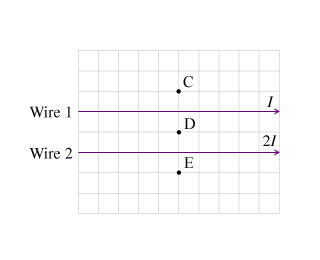# Problem: (a) What is the direction of the magnetic field at Point C? (b) What is the direction of the magnetic field at Point D? (c) What is the direction of the magnetic field at Point E?

###### FREE Expert Solution

Magnetic field around conductors:

$\overline{){\mathbf{B}}{\mathbf{=}}\frac{{\mathbf{\mu }}_{\mathbf{0}}\mathbf{i}}{\mathbf{2}\mathbf{\pi }\mathbf{r}}}$

Right-hand rule: Thumb points in the direction of the current. Fingers curl in the direction of the magnetic field.

(a)

At C, the field due to both conductors is directed in the same direction.

99% (104 ratings)###### Problem Details(a) What is the direction of the magnetic field at Point C?

(b) What is the direction of the magnetic field at Point D?

(c) What is the direction of the magnetic field at Point E?